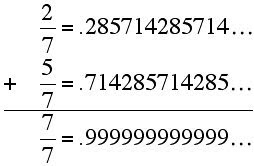## Monday, 4 August 2008

### Repeating Decimal Periods and PatternsIn my last post I asked, "IF you try to find the decimal number for 1/17 on the TI-84 it says 1/17 = .0588235294... and that's all you get. Now the challenge.... can you write out the complete decimal expansion (one complete cycle of the repeating expansion) of 1/17 using only what the calculator gave you and simple mental arithmetic...?"

The repeating portion of a decimal fraction is called the repetend, and the number of digits in the repeating cycle is called the period or length of the repetend. For 2/7, as shown above, the repetend is 285714 and has a length of 6. To figure out the original question I asked, it helps to know a rule about the length of repetends; for any prime number p > 5 the length of the repetend of 1/p is a factor of p-1. This seems first to have been stated by Johann H. Lambert in 1769. I find it odd that we had discovered so much about Primes so early, and this little tidbit came so late. For seven above, the length of 1/7 (or n/7) is six digits long. In looking at 1/17, the rule says that the period of the repetend must be a factor of 16, so the length will be 2, 4, 8, or 16 digits. Looking at what is given, we realize that the period can not be any of the smaller numbers, so it must have a 16 digit cycle, but how do we find the other digits?

Look back at 2/7 and another interesting property of repeating decimals may pop out at you. This one was published by Henry Goodwyn in 1802. He noted that if you divide the period into a first half and a second half, the sum of the digits in corresponding positions add up to nine.. For 2/7 above the repeat cycle is 285714 so the halfs are 285 and 714. Note that 2+7 = 8+1= 5+4 = 9, and Goodwyn showed this is true for ALL repeating decimals..... so it must be true for 1/17. If we know the period is 16 digits, then the first eight are 05882352 and to find the next eight, we just use the nines compliment of each number in the first eight, so they are 94117647, and we see that 1/17 = .0588235294117647...Now suppose we suddenly needed 2/17, we could double the string above, or just double the first half, and use the rule to complete the cycle.

In the age of computing when we can get out to a couple of hundred digits with almost any software, it might not seem like much of a tool....but sitting around drinking beers at the lake, and someone asks, "by the way, what is the repetend of 1/23?", you can whip out your pencil and do the first 11 digits and then coast the rest of the way adding nines-compliments.

I feel like I should have picked that one up somewhere in the seventh grade, but stumbled across it a few days ago reading an old book on Primes by the late George Loweke, who taught at Wayne State Univ. before retiring a few miles up the coast of Lake Michigan from me. Thanks George, but you should have mentioned this one to me sooner.Anonymous said...

I got a note on another link that pointed out that the statement above by Henry Godwin is not true as written.....

pat: Amazing! However, it doesn’t seem to be true for ALL repeating decimals. For example, it isn’t true for 1/21 = 0. or for 1/31 = 0..

I'm checking, but believe it may be true only for those decimals that repeat their full period (n-1 digits)...Anonymous said...

I found a link that explains this in more detail, the pdf is several pages, so I will just provide the link..
http://www.muskingum.edu/~rdaquila/m495/art/Repeating%20Decimals-Arledge.pdfAnonymous said...

If the period of a repeating decimal for k/p , where p is prime and k/p is a reduced fraction, has an even number of digits, then dividing the repeating portion into halves and adding gives a string of 9s. 1/21 doesn't apply because 21 is not prime, 1/31 does not work because the period is 15, not a multiple of two.Anonymous said...
This comment has been removed by a blog administrator.